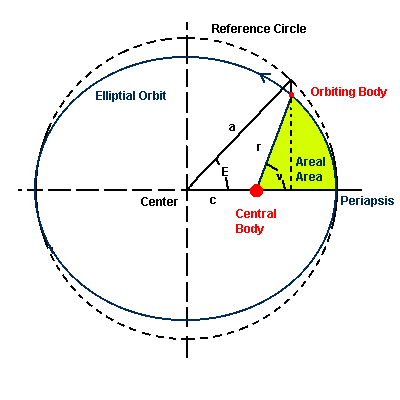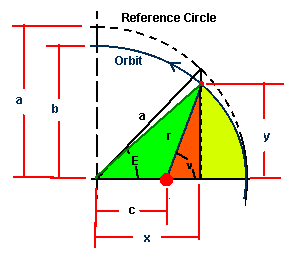## Determination of Position in Orbits

### Kepler's Equation and Eccentric AnomalyIntegration of the velocity in orbit is not easy. An easier method uses a reference circle center on the center of the elliptical orbit with a radius equal to the semi-major axis. The construction is shown here.

Equation of the Ellipse
x2/a2 + y2/b2 = 1
with origin at the center (not the focus) of the ellipse.
a = semi-major axis
b = semi-minor axis
a2 = c2 + b2 and c = e*a
with e = eccentricity of the elliptical orbit.
so.... b2 = a2(1 - e2)

Equation of the reference circle is
x2/a2 + y2/a2 = 1

### Eccentric Anamoly

If the position of the orbiting body is projected onto the reference circle as shown, the angular position of the reference position is called the eccentric anomaly, E. This parameter can be related to the area swept out by the radial line from the central body (focus of the elliptical orbit) to the orbiting body. The reference position when time = zero will be when the body is at periapsis.

The angular position of the body from periapsis as measured about the focus is the true anomaly, n.

Note that the y value in orbit is just equal to b/a = √(1 - e2) of the y value of the reference point on the reference circle.

Derivation of the relationship of the areal area to the eccentric anamoly, E, procedes as follows

1. Determine the area swept out by the radial line from the center to the object projection point on the reference circle (call it the reference area)
2. Reduce the reference area by the ratio of y values of the ellipse to the circle
3. Subtract off the extra triangular area due to the reference being to the center rather than the focus.The diagram to the right can be used for reference: The reference area after reduced is just the sum of the green, orange and yellow areas. The areal area is the orange plus yellow area. The green area has to be subtract away. (π = 3.1415926535..)

Area 1 = Reference Area = πa2 E/2π = E a2/2

Area 2 = Reference Area reduce by the ratio of ellipse height to reference circle height = green + orange + yellow regions = E a2√(1 - e2)/2

Area 3 = Area to be subtracted from Area 2 to give the Areal Area = green region = triangle area = 1/2 base x height = a sin(E) √(1 - e2) e a/2

Areal Area = Area 2 - Area 1 = E a2√(1 - e2)/2 - a2 e sin(E) √(1 - e2)/2 = [a2 √(1 - e2)/2][E - e sin(E)]

Note that the Areal Area has a relatively simple relationship with Eccentric Anomaly compared to that for the True Anomaly (an elliptical integral). This areal area is proportional to time. When the area equals the area of the ellipse, then the time is the period of the orbit, P.
Areal Area = K t = [a2 √(1 - e2)/2][E - e sin(E)]
Where t is time from periapsis and K is a proportionality constant to be determined
When E = 2π then Areal Area = πab = πa2 √(1 - e2)

The constant, K, can now be evaluated: K P = [a2 √(1 - e2)/2][2π] since sin(2π) = 0
but the area of the ellipse is the same area = πab = πa2 √(1 - e2)

So K = πa2 √(1 - e2)/P
πa2 √(1 - e2)/P = [a2 √(1 - e2)/2][E - e sin(E)]
2πt/P = E - e sin(E)

This is called Kepler's Equation and gives a direct relationship between time and position on the eccentric reference circle. It is relatively easy to determine True Anomaly from Eccentric Anomaly.

The quantity 2πt/P is called the Mean Anomaly and represent by the letter, M.

Kepler's Equation is usually seen written as
M = E - e sin(E)

### True Anomaly and Eccentric Anomaly

The x value of the position can be expressed in terms of both E and n.
x = a cos(E)
and
x = r cos(ν) + c = r cos(ν) + e a
where r is the equation for an ellipse with respect to the central body.
r = (1 - e2)a/(1 + e cos(ν))
So......

a cos(E) = [(1 - e2)cos(ν)/(1 + e cos(ν))]a + e a

cos(E)= [(1 - e2)cos(ν)/(1 + e cos(ν))] + e
OR
cos(E) = [cos(ν) + e]/[1 + e cos(ν)]

Solving for cos(ν) in terms of cos(E) gives:
cos(ν) = [cos(E) - e]/[1 - e cos(E)]